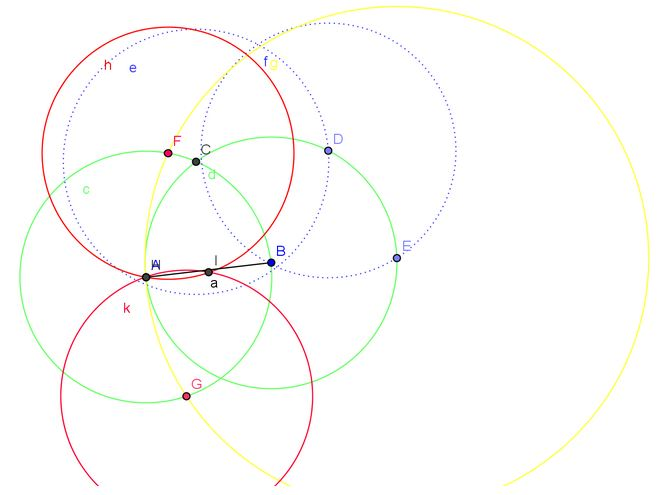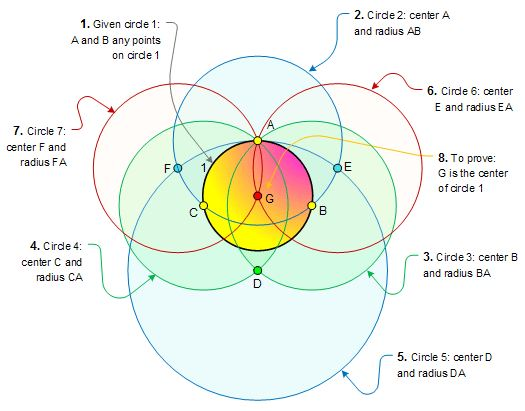Geometric Transformations IV: Circular Transformations 应该有一个中文译本, 最主要的理由是:

• 这是一本非常精彩的书, 很经典;
• 读者主要是中学师生.

I. M. Yaglom(Isaak Moiseevich Yaglom, March 6, 1921 –  April 7, 1988) 的 Geometric Transformations 的第四册是 Geometric Transformations IV: Circular Transformations.

Geometric Transformations (几何变换)是前苏联数学家 I. M. Yaglom 的经典著作, 内容分为三部分, 作两册出版: 前两部分为第一册, 第三部分为第二册. 美国数学会在 $$1960$$ 年代的”新数学”运动期间, 出版了一套”新数学丛书”, 其中就有这本 Geometric Transformations. 英文版分为四册, 前三册分别于 $$1962, 1968, 1973$$ 年出版, 但第四册迟迟没有下文, 直到 $$2009$$ 年, 才出版了第四册, 就是本文的主角, 这离第三册的面世已经过去整整 $$36$$ 个年头.

Geometric Transformations 大概是最系统的论述初等几何的几何变换的著作. 具体来说, 初等几何中, 除去那些具体的定理之外, 还有两个重要的有普遍意义的, 构成了几何学的一切进一步发展的基础, 的思想, 其重要性远远超出了几何学的界限. 这两个思想的一个是演绎法和几何学的公理基础, 另一个是几何变换和几何学的群论基础. 本书是阐述后者–几何变换和几何学的群论基础–的.

Yaglom 于 $$1945$$ 年获得国立Moscow大学的博士学位. 他写了超过 $$40$$ 本书和一些文章, 有好几本著作被译成了英文, 已经是标准的学术参考书.

• Author: I.M. Yaglom (translated by A. Shenitzer)
• Paperback: 285 pages
• Publisher: Mathematical Association of America (September 14, 2009)
• Language: English
• ISBN-10: 0883856484
• ISBN-13: 978-0883856482

## 仅用圆规找中点1. 分别以 $$A,B$$ 为圆心, $$AB$$ 为半径画圆(绿色)交于 $$C$$;
2. 在圆 $$B$$ 上取 $$D,E$$ 两点, 使 $$CD=DE=AB$$, 则 $$AE=2AB$$;
3. 以 $$E$$ 为圆心,  $$AE$$ 为半径画圆(黄色)交圆 $$A$$ 于  $$F,G$$;
4. 分別以 $$F,G$$ 为圆心, $$AF$$ 为半径画圆(红色), 交于 $$A,I$$ 两点, 则 $$I$$ 就是 $$AB$$ 中点.

## 仅用圆规找圆心

$$\color{red}O$$ 给定仅仅使用圆规找出圆心.

1. 在圆 $$O$$ 上任取一点 $$A$$,以 $$A$$ 为圆心画圆, 交圆 $$O$$ 于 $$B,C$$ 兩点;
2. 分別以 $$B,C$$ 为心,$$AB$$ 为半径画圆交于 $$D$$ 点;
3. 以 $$D$$ 为圆心, $$AD$$ 为半径画圆交圆 $$A$$ 于 $$E,F$$ 两点;
4. 再分别以 $$E,F$$ 为圆心,$$AE$$ 为半径画圆,交于 $$A,G$$, 则 $$G$$ 就是 $$O$$ 点.1. 在已知圆周上任取一点 $$A$$, 以 $$A$$ 为圆心, 适当长为半径作圆 $$A$$, 交已知圆于两点 $$B,C$$;
2. 从 $$B$$ 点出发, 以 $$AB$$ 长为半径, 在圆 $$A$$ 上连续截取 $$3$$ 次得到点 $$D$$;
3. 分别以 $$A,D$$ 为圆心,$$CD$$ 为半径作弧, 两弧交于 $$E$$;
4. 再以 $$E$$ 为圆心, $$EA$$ 为半径作弧交圆 $$A$$ 于 $$F$$;
5. 分别以 $$A,B$$ 为圆心, $$FB$$ 为半径作弧, 两弧的交点就是所求已知圆的圆心.